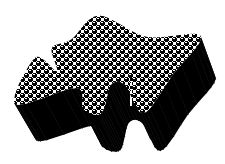# Definition Of Mass

## Definition Of Mass

"The amount of matter contained in an object" is known as the mass of the object.
If there are "n" particles in an object, then the sum of individual masses of "n" particles is known as mass of the object.Mass is the fundamental property of an object. (Fundamental quantities - length, mass and time. All other physical quantities like speed, velocity, weight can be defined in terms of length, mass and time).
Mass is an unchanging property of an object. (Mass of the body never changes from place to place)
Mass is denoted by letter �m�
The S.I. unit of mass is Kilogram
Mass is measured with beam balance.
If a body has a mass of 60 Kg on earth, even on the moon the mass of the same body is 60 Kg.
Weight changes from places to place. (Commonly we are often confused with the terms mass and weight, weight of the object is different to that of mass of the object).
Weight is the force with which body is attracted towards earth. Weight is the product of mass and acceleration due to gravity (g). W = mg.
If mass of an object is 10 Kg then the weight is 100 Newtons. (Weight is measured in newtons.)
When an object is at rest, we need force to make the object to move. In the same way we need force to stop the moving object. Objects generally oppose (resist) change in its state. This property is known as inertia.
Mass is the measure of inertia. The more the mass of object, more force is required to change its state (Rest-Motion or Motion-rest).

#### Worked Example:

Example 1: Which of the following quantity is measured in Kilogram?

A. Weight
B. Force
C. Mass
D. Acceleration
The S.I. unit of mass is Kilogram

Example 2: If a body has a mass of 3000Kg on earth, what is its mass on the surface of the planet mercury?

A. 1000Kg
B. 2000Kg
C. 3000Kg
D. 4000Kg
The mass of the object will never change from one place to another place. Its value is constant throughout the universe.

Example 3: Which of the following property of a body cannot be changed by applying force on the object?

A. Shape of the body.
B. Size of the body.
C. Weight of the body.
D. Mass of the body.